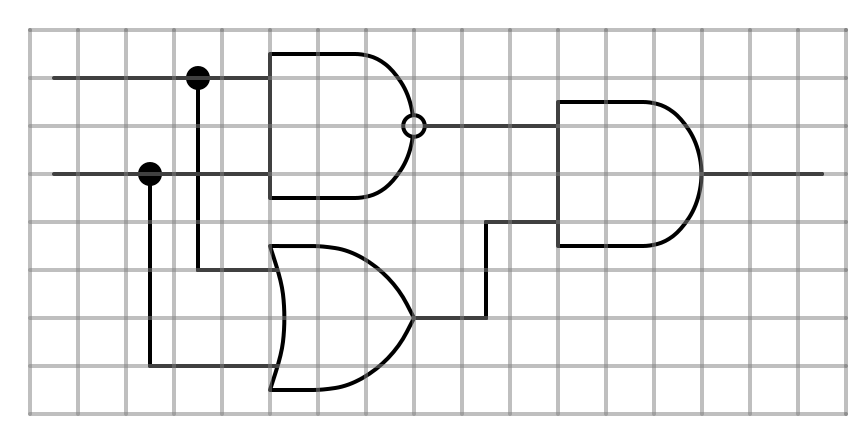8.2

## Diagrama: A diagram drawing library

Digrama is a library for drawing diagrams on top of pict.

### 1Diagrama API Reference

 (require diagrama) package: diagrama-lib

Warning: The API presented here is unstable, and may change without warning.

 procedure(diagram? it) → boolean? it : any/c
Is it a Diagram. Diagrams are computations that draw, well, diagrams. Diagrams have a state which consists of a current drawing location, a notion of units, a line-width, and line-color.

Diagrams are pict-convertible?. When diagrams are drawn the whole image is shifted such that the minimum x and y coordinate are shifted to the origin. When diagrams are converted to pict?s the starting coordinates are always (0,0).

#### 1.1Basic diagram constructors

 value
An empty diagram.

 procedure(img p [align]) → diagram? p : pict-convertible? align : (or/c 'lt 'ct 'rt 'lc 'cc 'rc 'lb 'cb 'rb) = 'cc
Convert this p into a diagram which just draws p. The part of p designated by align controls which part of p is placed at the current location. For example 'cc centers it.

 procedure(path path [fill-style]) → diagram? path : (is-a?/c dc-path%) fill-style : (or/c 'odd-even 'winding) = 'odd-even
Draw the given path. The path is interpreted in terms of the current location and units, and the current location after drawing the path is the location of the last point in the paths. The path is not mutated.

The fill-style is the same as the same argument from draw-path.

Examples:
 > (define unit-line-right (let () (define p (new dc-path%)) (send p move-to 0 0) (send p line-to 1 0) (path p)))
> unit-line-right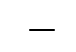> (after (move-to 3 0) unit-line-right)> (after (units 36) (move-to 3 0) unit-line-right)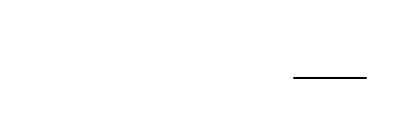> (after (units 36) (move-to 3 0) (color "red") unit-line-right)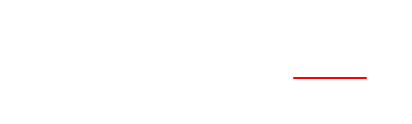procedure(line-to x y [#:h-first h-fit]) → diagram? x : real? y : real? h-fit : any/c = #t
Creates a diagram which draws a line from the current location to (x,y). The line moves only horizontally and vertically. If h-first is not #f it moves horizontally then vertically, otherwise it does the reverse.

Example:
 > (line-to 3 2)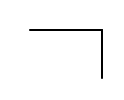procedure d : real?
 procedure d : real?
 procedure(line-up d) → diagram? d : real?
 procedure d : real?
Create a diagram which draws a line from the current location d away in the given direction.

Examples:
 > (line-right 5)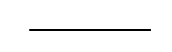> (line-up 5)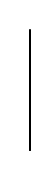procedure(move-to x y) → diagram? x : real? y : real?
Makes an empty diagram which moves the current drawing location to (x,y), with (0,0) being in the upper left.

Example:
 > (after (move-to 3 3) (line-right 5))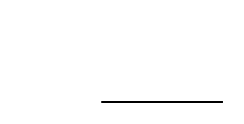procedure d : real?
 procedure d : real?
 procedure(move-up d) → diagram? d : real?
 procedure d : real?
Makes an empty diagram which moves the current location by d in the corresponding direction.

 procedure(tag-location name [x y]) → diagram? name : any/c x : real? = #f y : real? = #f
Make an empty diagram that names ta location name. If x and y given that location is named, otherwise the current location is names. This will overwrite any existing locations which have a name equal? to name.

 procedure(move-to-tag name) → diagram? name : any/c
Move to the location with the given name. Errors if no location has that name.

 procedure(line-to-tag name [#:h-first h-fit]) → diagram? name : any/c h-fit : any/c = #t
Draw a line from the current location to the location with the given name. Errors if no location has that name. The line is drawn like line-to.

Example:
 > (after (tag-location 'here 3 3) (move-to 0 0) (line-to-tag 'here))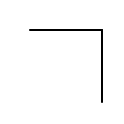procedure(line-between start end [#:h-first h-fit]) → diagram start : any/c end : any/c h-fit : any/c = #t
Draw a line between the two named coordinates. See also line-to-tag and line-to.

Example:
 > (after (tag-location 'here 1 2) (tag-location 'there 4 0) (line-between 'here 'there))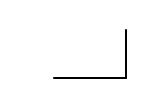procedure(units u) → diagram? u : positive?
Create an empty diagram that changes the current size of the coordinate system to u. The default is 12.

Examples:
 > (define l (line-right 1)) > l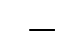> (after (units 36) l)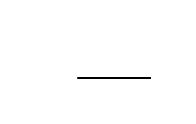procedure(color c) → diagram? c : (or/c string? (is-a?/c color%))
Create an empty diagram that changes the current line color.

Example:
 > (after (units 36) (color "red") (line-right 2))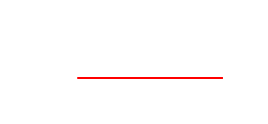procedure l : (real-in 0 255)
Create an empty diagram that changes the current line width.

 procedure(label t dir) → diagram? t : string? dir : (or/c 'up 'down 'left 'right)
Add text to the diagram one unit in the given direction.

Example:
 > (after (units 24) (save (label "Line" 'right)) (line-up 1) (line-down 2))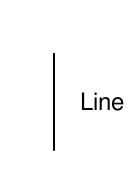value
Draws a grid over the current diagram with length/width of each cell of unit length.

Examples:
> (define l (line-right 3))
> l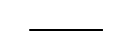> (after l unit-grid)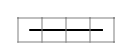> (after l (units 24) unit-grid)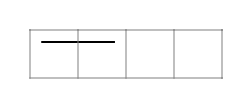> (after (units 24) l unit-grid)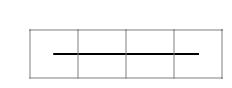> (after (save l) (move-down 1) (save l) (move-down 1) (save l) unit-grid)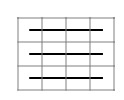#### 1.2Diagram composition

 procedure(after d ...) → diagram? d : diagram?
Draw all of the ds one after another.

Example:
 > (after (line-up 3) (line-right 3) (line-down 3) (line-left 3))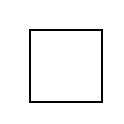procedure(before d1 d ...) → diagram? d1 : diagram? d : diagram?
Draw all of the ds one after another, then draw d1 at with initial state of the diagram.

Examples:
 > (after (img (disk 36 #:color "white")) (line-right 3))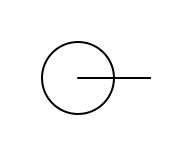> (before (img (disk 36 #:color "white")) (line-right 3))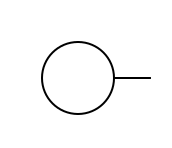procedure(save d ...) → diagram? d : diagram?
Draw all of d one after another, then resort the current units and location to what they were at the start of the save.

 procedure(save/bounds d ...) → diagram? d : diagram?
Like save except the bounds of the diagram are are restored after the ds are draw. This allows for drawing outside of the bounds of the resulting pict?.

 procedure(split d1 d2) → diagram? d1 : diagram? d2 : diagram
Draw d1 and d2 with the current state, and place a black dot at the current location. The resulting state is the state from d2.

Example:
 > (after (line-right 3) (split (after (line-up 3) (line-right 3)) (after (line-down 3) (line-right 3))) (line-down 3))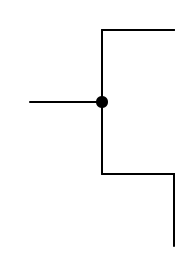procedure(*> d1 d ...) → diagram? d1 : diagram? d : diagram?
Draw all of the diagrams in order, with each being drawn with the initial state. The resulting state is that of the last diagram.

Example:
 > (after (units 36) (*> (line-up 1) (line-down 1) (line-left 1) (line-right 1)) (line-down 1))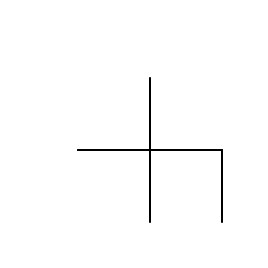procedure(<* d1 d ...) → diagram? d1 : diagram? d : diagram?
Draw all of the diagrams in order, with each being drawn with the initial state. The resulting state is that of the first diagram.

Example:
 > (after (units 36) (<* (line-up 1) (line-down 1) (line-left 1) (line-right 1)) (line-right 1))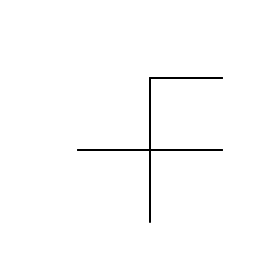procedure(start-at #:ud ud #:lr lr d ...) → diagram ud : (or/c 'up 'down) lr : (or/c 'left 'right) d : diagram?
Draw the diagrams in order, with each starting at the location on the corner of the previous specified by ud and lr. The first diagram is drawn at the current location.

 syntax(for/after (for-clauses ...) body-or-break ... body)
 syntax(for*/after (for-clauses ...) body-or-break ... body)
 syntax(for/*> (for-clauses ...) body-or-break ... body)
 syntax(for*/*> (for-clauses ...) body-or-break ... body)
 syntax(for/<* (for-clauses ...) body-or-break ... body)
 syntax(for*/<* (for-clauses ...) body-or-break ... body)
Your friendly neighborhood for forms, for building diagrams using after, *>, and <*.

#### 1.3Reflecting on the drawing state

There are several ways to directly inspect the current drawing state. These are all fairly low level operations that are most likely useful for making new combinators, or when making pict?’s that scale to the current unit size (for example unit-grid and start-at are defined with these).

 procedure(with-loc builder) → diagram? builder : (-> real? real? diagram?)
Build a diagram using the current (x,y) location.

 procedure(with-bounds builder) → diagram? builder : (-> real? real? real? real? diagram?)
Build a diagram given the current bounding box. See with-state for the order of arguments to builder.

 procedure(with-color builder) → diagram? builder : (-> (or/c string? (is-a?/c color%)) diagram?)
Build a diagram using the current color.

 procedure(with-line-width builder) → diagram? builder : (-> (real-in 0 255) diagram?)
Build a diagram using the current line width.

 procedure(with-unit builder) → diagram? builder : (-> real? diagram?)
Build a diagram given the current units.

Examples:
 > (define unit-circle (with-unit (compose img circle)))
> unit-circle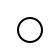> (after (units 24) unit-circle)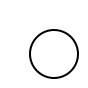> (scale (after (units 24) (for*/fold ([p nothing]) ([x (in-range 3)] [y (in-range 3)]) (after p (move-to x y) unit-circle)) unit-grid) 2)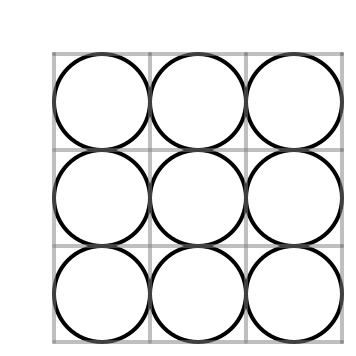procedure(with-location-of tag builder) → diagram? tag : any/c builder : (-> real? real? diagram?)
Build a diagram using the the given named location.

#### 1.4Circuit Helpers

 (require diagrama/circuit) package: diagrama-lib

diagrama/circuit has helpers for drawing circuit diagrams. Note that it is easy to accidentally draw lines on top of gates: before is designed to help with this.

procedure

 (or-gate [ #:in1 n1 #:in2 n2 #:in3 n3 #:out out #:tag-in1 tag1 #:tag-in2 tag2 #:tag-in3 tag3 #:tag-out tag4]) → diagram?
n1 : any/c = #f
n2 : any/c = #f
n3 : any/c = #f
out : any/c = #f
tag1 : any/c = #f
tag2 : any/c = #f
tag3 : any/c = #f
tag4 : any/c = #f

procedure

 (and-gate [ #:in1 n1 #:in2 n2 #:in3 n3 #:out out #:tag-in1 tag1 #:tag-in2 tag2 #:tag-in3 tag3 #:tag-out tag4]) → diagram?
n1 : any/c = #f
n2 : any/c = #f
n3 : any/c = #f
out : any/c = #f
tag1 : any/c = #f
tag2 : any/c = #f
tag3 : any/c = #f
tag4 : any/c = #f

procedure

 (buffer [ #:in2 n2 #:out out #:tag-in2 tag2 #:tag-out tag4]) → diagram?
n2 : any/c = #f
out : any/c = #f
tag2 : any/c = #f
tag4 : any/c = #f

procedure

 (register [ #:in2 n2 #:out out #:tag-in2 tag2 #:tag-out tag4]) → diagram?
n2 : any/c = #f
out : any/c = #f
tag2 : any/c = #f
tag4 : any/c = #f
Make a diagram that draws the given gate, each gate facing to the right. or-gate and and-gate are three units square, and designed to take in up to three input wires. buffer and register are roughly the same size, but are designed to take only one input. If n1, n2, or n3 are not #f, then the upper, middle, or lower input (respectively) are negated. out does the same for the output. If tag1, tag2 or tag3 a wire is drawn for those inputs, and its endpoint is named by the given tag. tag4 does the same for the output.

The drawn gate is centered at the current location.

Examples:
> (define layer-1-x 6)
> (define layer-2-x 3)
> (define input-1 (tag-location 'input-1 0 0))
> (define input-2 (tag-location 'input-2 0 2))
 > (define top-gate (after (move-down 1) (move-right layer-1-x) (and-gate #:out #t #:tag-out 'and-out #:tag-in1 'and-A #:tag-in3 'and-B)))
 > (define lower-gate (after (move-down 5) (move-right layer-1-x) (or-gate #:tag-out 'or-out #:tag-in1 'or-A #:tag-in3 'or-B)))
 > (define last-gate (after (move-down 1) (move-right layer-2-x) (and-gate #:tag-in1 'and-in #:tag-in3 'or-in #:tag-out 'result)))
 > (define (connect-input input g1 g2 split-point) (after (move-to-tag input) (line-right split-point) (split (line-to-tag g1) (line-to-tag g2 #:h-first #f))))
 > (define xor (after input-1 input-2 (move-to-tag 'input-1) (save top-gate) (save lower-gate) (move-to-tag 'and-out) last-gate (connect-input 'input-1 'and-A 'or-A 3) (connect-input 'input-2 'and-B 'or-B 2) (line-between 'and-out 'and-in) (line-between 'or-out 'or-in) (move-to-tag 'result) (line-right 1)))
> (scale xor 2)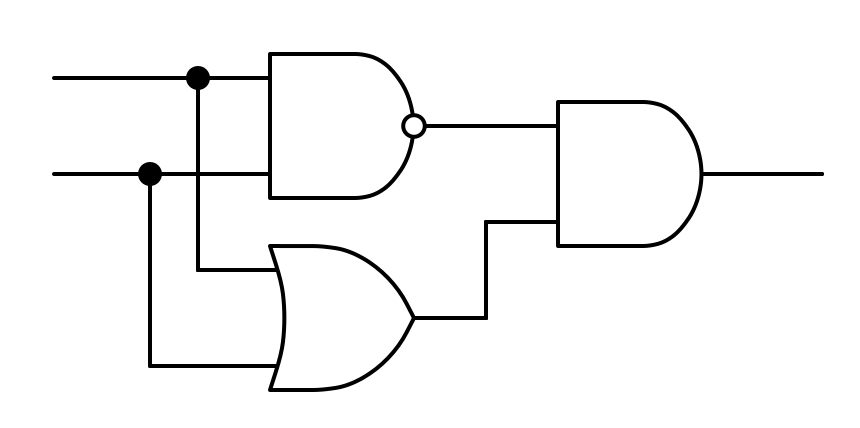> (scale (after xor unit-grid) 2)